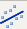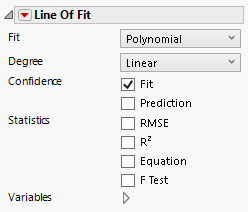Publication date: 05/24/2021

## Line of Fit

The Line of Fit elementshows a linear regression line with confidence intervals for the fit.

Tip: To label a line of fit, right-click on the line element in the legend.

Figure 3.27 Line of Fit OptionsFit

Specifies the type of fit:

Polynomial

A simple linear regression.

Robust Cauchy

A linear regression assuming Cauchy distributed residuals, to de-emphasize outliers.

Time Series

Smoothing for equally spaced X values with optimal seasonality. Includes options for a forecast model and the number of seasonal and forecast periods. The smoothing model is selected from a subset of state space smoothing models defined by Hyndman et al. (2008). See Modeling Specifications in Predictive and Specialized Modeling.

Degree

Specifies the polynomial degree of the linear regression fit, which can be linear, quadratic, or cubic.

Confidence

Shows or hides confidence intervals for the predicted value (Fit) or for individual values (Prediction). Both types of intervals are fixed at 95% confidence.

Statistics

Shows various selections on the graph. You can show the root mean square error (RMSE), R-square, the equation of the regression line, and the F Test value.

Variables

Shows or hides graph elements for variables, or re-orders the display of variables.

Note: These options do not apply to variables in the Group X, Group Y, Wrap, or Page zones.

Check boxes are followed by the zone designation and the name of the variable. Use check boxes to do the following:

Show or hide the elements corresponding to a variable in a zone.

Add or remove the effect of applying the Color, Size, Shape, or Freq variable to the variable in the zone.

Tip: If you have multiple graphs, you can color or size each graph by different variables. Drag a second variable to the Color or Size zone, and drop it in a corner. In the Variables option, select the specific color or size variable to apply to each graph.

Use arrows to re-order the display if there are multiple variables in a zone. Highlight a variable name and click an arrow to reposition it.

For an example using Variables, see Example of an Area and Line Chart.

#### Red Triangle Options for Line of Fit

Response Axis

Specifies the axis for the variable that is used as the response in the calculation of the linear regression line. Because standard least squares typically uses the Y (vertical) axis as the response axis, the Auto setting defaults to the Y axis.